• ## 隐函数求导公式

万次阅读 2018-04-22 08:42:34
隐函数存在定理1： 设函数F(x,y)F(x,y)F(x,y)在点P(x0,y0)P(x0,y0)P(x_0,y_0)的某一邻域内具有连续的偏导数，且F(x0,y0)=0,Fx(x0,y0)≠0F(x0,y0)=0,Fx(x0,y0)≠0F(x_0,y_0)=0,F_x(x_0,y_0) \neq 0，则方程F(x,y)=0F...
一、单方程形式
隐函数存在定理1：  设函数

F(x,y)

F

(

x

,

y

)

$F(x,y)$在点

P(x0,y0)

P

(

x

0

,

y

0

)

$P(x_0,y_0)$的某一邻域内具有连续的偏导数，且

F(x0,y0)=0,Fx(x0,y0)≠0

F

(

x

0

,

y

0

)

=

0

,

F

x

(

x

0

,

y

0

)

≠

0

$F(x_0,y_0)=0,F_x(x_0,y_0) \neq 0$，则方程

F(x,y)=0

F

(

x

,

y

)

=

0

$F(x,y)=0$在点

(x0,y0)

(

x

0

,

y

0

)

$(x_0,y_0)$的某一邻域内恒能唯一确定一个连续且具有连续导数的函数

y=f(x)

y

=

f

(

x

)

$y=f(x)$，它能满足条件

y0=f(x0)

y

0

=

f

(

x

0

)

$y_0=f(x_0)$，并有

dydx=−FxFy（负分式交叉对应）

d

y

d

x

=

−

F

x

F

y

（

负

分

式

交

叉

对

应

）

\frac{dy}{dx} = -\frac{F_x}{F_y}（负分式交叉对应）

隐函数存在定理2：  设函数

F(x,y,z)

F

(

x

,

y

,

z

)

$F(x,y,z)$在点

P(x0,y0,z0)

P

(

x

0

,

y

0

,

z

0

)

$P(x_0,y_0,z_0)$的某一邻域内具有连续的偏导数，且

F(x0,y0,z0)=0,Fx(x0,y0,z0)≠0

F

(

x

0

,

y

0

,

z

0

)

=

0

,

F

x

(

x

0

,

y

0

,

z

0

)

≠

0

$F(x_0,y_0,z_0)=0,F_x(x_0,y_0,z_0) \neq 0$，则方程

F(x,y,z)=0

F

(

x

,

y

,

z

)

=

0

$F(x,y,z)=0$在点

(x0,y0,z0)

(

x

0

,

y

0

,

z

0

)

$(x_0,y_0,z_0)$的某一邻域内恒能唯一确定一个连续且具有连续导数的函数

z=f(x,y)

z

=

f

(

x

,

y

)

$z=f(x,y)$，它能满足条件

z0=f(x0,y0)

z

0

=

f

(

x

0

,

y

0

)

$z_0=f(x_0,y_0)$，并有

dzdx=−FxFz

d

z

d

x

=

−

F

x

F

z

\frac{dz}{dx} = -\frac{F_x}{F_z}

dzdy=−FyFz

d

z

d

y

=

−

F

y

F

z

\frac{dz}{dy} = -\frac{F_y}{F_z}

二、方程组形式
若考虑方程组

{F(x,y,u,v)=0G(x,y,u,v)=0

{

F

(

x

,

y

,

u

,

v

)

=

0

G

(

x

,

y

,

u

,

v

)

=

0

\begin{cases}
F(x,y,u,v)=0 \\
G(x,y,u,v)=0
\end{cases}其中

u、v是关于x、y的函数

u

、

v

是

关

于

x

、

y

的

函

数

$u、v是关于x、y的函数$

若要求

∂u∂x、∂v∂x

∂

u

∂

x

、

∂

v

∂

x

$\frac{\partial u}{\partial x}、\frac{\partial v}{\partial x}$  则

F(x,y,u,v)=0、G(x,y,u,v)=0

F

(

x

,

y

,

u

,

v

)

=

0

、

G

(

x

,

y

,

u

,

v

)

=

0

$F(x,y,u,v)=0、G(x,y,u,v)=0$的两边对

x

x

$x$求导得{−Fx=Fu∂u∂x+Fv∂v∂x−Gx=Gu∂u∂x+Gv∂v∂x$\left\{\begin{array}{l}-{F}_{x}={F}_{u}\frac{\mathrm{\partial }u}{\mathrm{\partial }x}+{F}_{v}\frac{\mathrm{\partial }v}{\mathrm{\partial }x}\\ -{G}_{x}={G}_{u}\frac{\mathrm{\partial }u}{\mathrm{\partial }x}+{G}_{v}\frac{\mathrm{\partial }v}{\mathrm{\partial }x}\end{array}$\begin{cases}
-F_x=F_u\frac{\partial u}{\partial x}+F_v \frac{\partial v}{\partial x}\\
-G_x=G_u\frac{\partial u}{\partial x}+G_v \frac{\partial v}{\partial x}
\end{cases}  化为行列式得：

D=∣∣∣FuGuFvGv∣∣∣

D

=

|

F

u

F

v

G

u

G

v

|

D=\begin{vmatrix}
F_u & F_v \\
G_u & G_v \\
\end{vmatrix}由范德蒙行列式的性质可得：

Du=∣∣∣−Fx−GxFvGv∣∣∣，Dv=∣∣∣FuGu−Fx−Gx∣∣∣

D

u

=

|

−

F

x

F

v

−

G

x

G

v

|

，

D

v

=

|

F

u

−

F

x

G

u

−

G

x

|

D_u=\begin{vmatrix}
-F_x & F_v \\
-G_x & G_v \\
\end{vmatrix}，D_v=\begin{vmatrix}
F_u & -F_x \\
G_u & -G_x \\
\end{vmatrix}可得：

∂u∂x=DuD，∂v∂x=DvD

∂

u

∂

x

=

D

u

D

，

∂

v

∂

x

=

D

v

D

\frac{\partial u}{\partial x} = \frac{D_u}{D}，\frac{\partial v}{\partial x} = \frac{D_v}{D}

展开全文高等数学
•文档
• 多元隐函数求导方法 一、一个方程的情形 1.F(x,y)=0 隐函数存在定理 1 设函数F(x, y)在点P(x0,y0)的某一邻域内具有连续的偏导数（条件1），且 F(x0,y0)=0 F(x0,y0)=0 F(x0,y0)=0 Fy′(x0,y0)≠0 F_{y}^{'} (x0,y0)\...


多元隐函数求导方法
一、一个方程的情形

1.F(x,y)=0
隐函数存在定理 1 设函数F(x, y)在点P(x0,y0)的某一邻域内具有连续的偏导数（条件1），且

F

(

x

0

,

y

0

)

=

0

F(x0,y0)=0

F

y

′

(

x

0

,

y

0

)

≠

0

F_{y}^{'} (x0,y0)\ne0

（条件2）则方程F(x, y) = 0在点 ( , ) 0 0 P x y 的 某一邻域内恒能唯一确定一个单值连续且具有连续导数的函数y = f (x)，它满足条件 ,并有

d

y

d

x

=

−

F

x

′

F

y

′

\frac{dy}{dx}=-\frac{F_{x}^{'}}{F_{y}^{'} }

​ 以上同时为隐函数的求导公式为
F(x, y, z) = 0 隐函数存在定理 2 设函数F(x, y, z)在点 P(x0,y,z0)某一领域内有连续的偏导数（条件1），且F(x0,y,z0)=0，

F

z

’

（

x

0

,

y

0

,

z

0

)

≠

0

F_{z}^{’}（x0,y0,z0)\ne 0

（条件2）则方程F(x, y,z)=0在点P(x0,y0,z0)的某一领域内恒有唯一确定一个单值连续且具有连续偏导数的函数z=f（x,y),它满足条件z0=f（x0，y0），并有

∂

z

∂

x

=

−

F

x

′

F

z

′

\frac{\partial z }{\partial x}=-\frac{F_{x}^{'} }{F_{z}^{'} }

∂

z

∂

y

=

−

F

y

′

F

z

′

\frac{\partial z }{\partial y}=-\frac{F_{y}^{'} }{F_{z}^{'} }

对于求二次偏导，可以使用两种该方法：1、等式两边同时求偏导，利用类似一元隐函数的隐函数求导。 2、利用上文展示的求导公式。 warning 在解法一中，我们在对x和y求偏导时，仍要将他们看作是z的自变量，亦指将z看作是（x，y）的二元函数； 在解法二中，我们求F（x,y,z)的三个偏导数时要注意，要将xyz看作独立的自变量。
​ 3、对于一些式子还可以对于等式两边取微分。
二、方程组的情形

{

F

(

x

,

y

,

u

,

v

)

=

0

G

(

x

,

y

,

u

,

v

)

=

0

\left\{\begin{matrix} F(x,y,u,v)=0 \\ G(x,y,u,v)=0 \end{matrix}\right.

隐函数存在定理3 设F(x, y,u,v)、G(x, y,u,v)在 点P（x0,y0,u0,v0)的某一领域内有对各个变量的连续偏导数（条件1，//可见连续偏导是最高级条件），且F（x0,y0,u0,v0)=0，G（x0,y0,u0,v0)=0,（条件2）且偏导数所组成的函数行列式（雅可比式）

J

=

∂

(

F

,

G

)

∂

(

u

,

v

)

=

∣

∂

F

∂

u

∂

F

∂

v

∂

G

∂

u

∂

G

∂

v

∣

J=\frac{\partial (F,G)}{\partial (u,v)}=\begin{vmatrix} \frac{\partial F}{\partial u}&\frac{\partial F}{\partial v} \\ \frac{\partial G}{\partial u}&\frac{\partial G}{\partial v} \end{vmatrix}

在P点函数值不等于零（条件3），则方程组在P 的某一领域内恒有唯一确定一组单值连续且具有连续偏导数的函数u=u(x,y),v=v(x,y),且将P点带入，函数成立（条件4//与条件2类似，指符合P点要求）。
​ 对于偏导数的求值，应当采用推导证明法。
例：

{

F

(

x

,

y

,

u

,

v

)

=

0

G

(

x

,

y

,

u

,

v

)

=

0

\left\{\begin{matrix} F(x,y,u,v)=0 \\ G(x,y,u,v)=0 \end{matrix}\right.

有隐函数组：

{

v

=

v

(

x

,

y

)

u

=

u

(

x

,

y

)

\left\{\begin{matrix} v=v(x,y) \\ u=u(x,y) \end{matrix}\right.

则：

{

F

(

x

,

y

,

u

(

x

,

y

)

,

v

(

x

,

y

)

)

≡

0

G

(

x

,

y

,

u

(

x

,

y

)

,

v

(

x

,

y

)

)

≡

0

\left\{\begin{matrix} F(x,y,u(x,y),v(x,y))\equiv 0\\ G(x,y,u(x,y),v(x,y))\equiv 0 \end{matrix}\right.

两边对于x求导

{

F

x

+

F

u

⋅

∂

u

∂

x

+

F

v

⋅

∂

v

∂

x

=

0

G

x

+

G

u

⋅

∂

u

∂

x

+

G

v

⋅

∂

v

∂

x

=

0

\left\{\begin{matrix} F_{x}+F_{u}\cdot \frac{\partial u}{\partial x}+F_{v}\cdot \frac{\partial v}{\partial x}=0 \\ G_{x}+G_{u}\cdot \frac{\partial u}{\partial x}+G_{v}\cdot \frac{\partial v}{\partial x}=0 \end{matrix}\right.

这是

∂

v

∂

x

，

∂

v

∂

x

\frac{\partial v}{\partial x}，\frac{\partial v}{\partial x}

的线性方程组，对于这个线性方程组运用克拉默法则，求解。
展开全文线性代数
• 隐函数求导公式(多元隐函数存在定理) 全微分 先代后求 全微分 多元函数求极值 交换积分次序
隐函数求导公式(多元隐函数存在定理)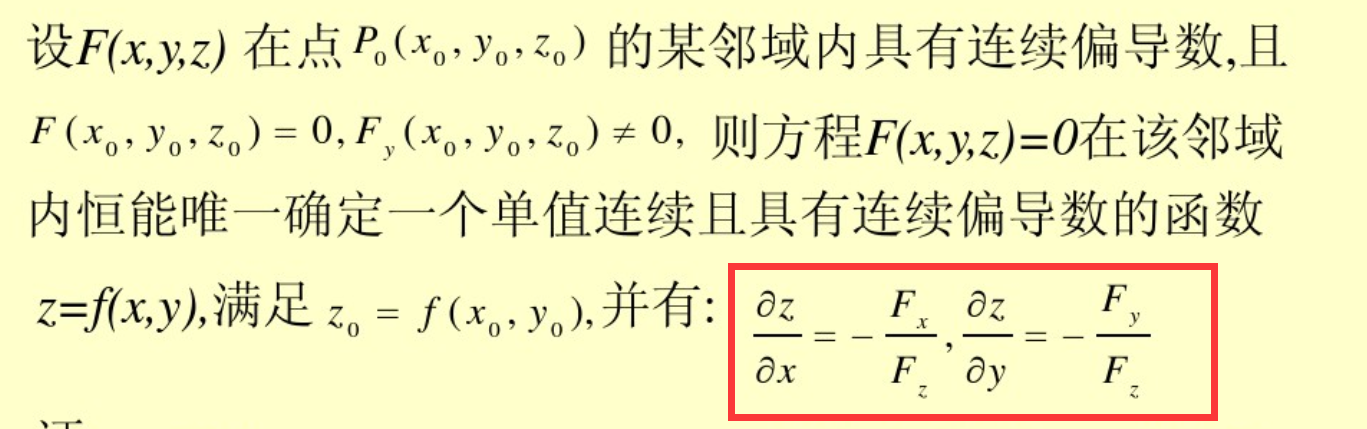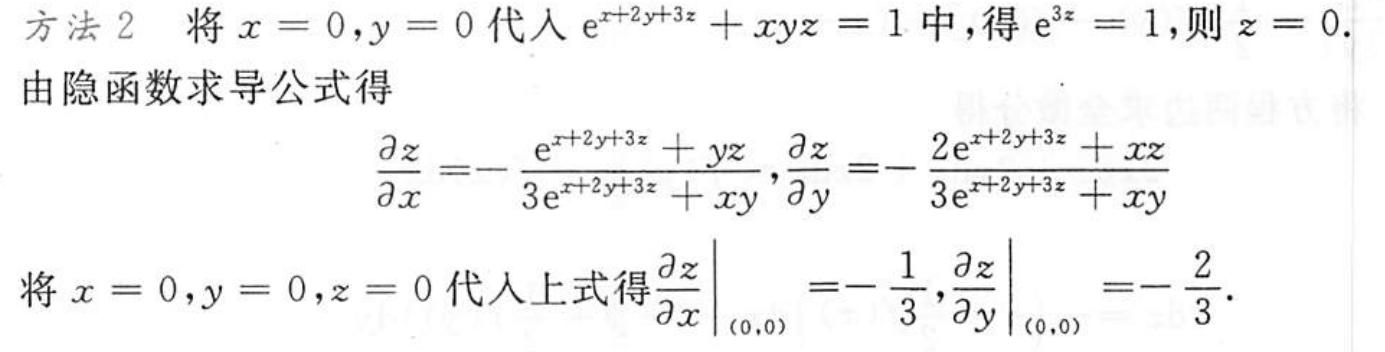全微分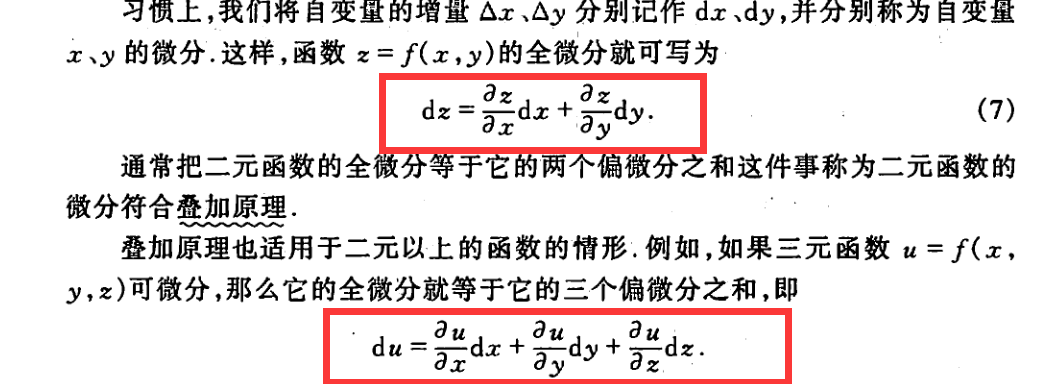先代后求 全微分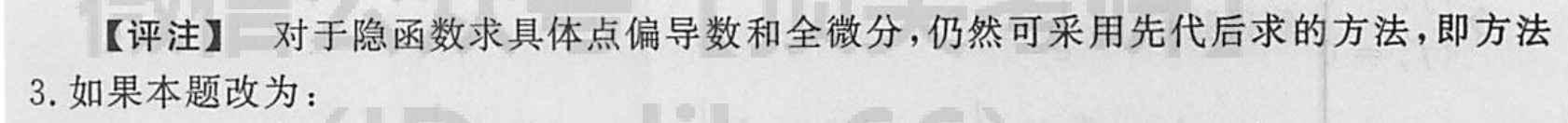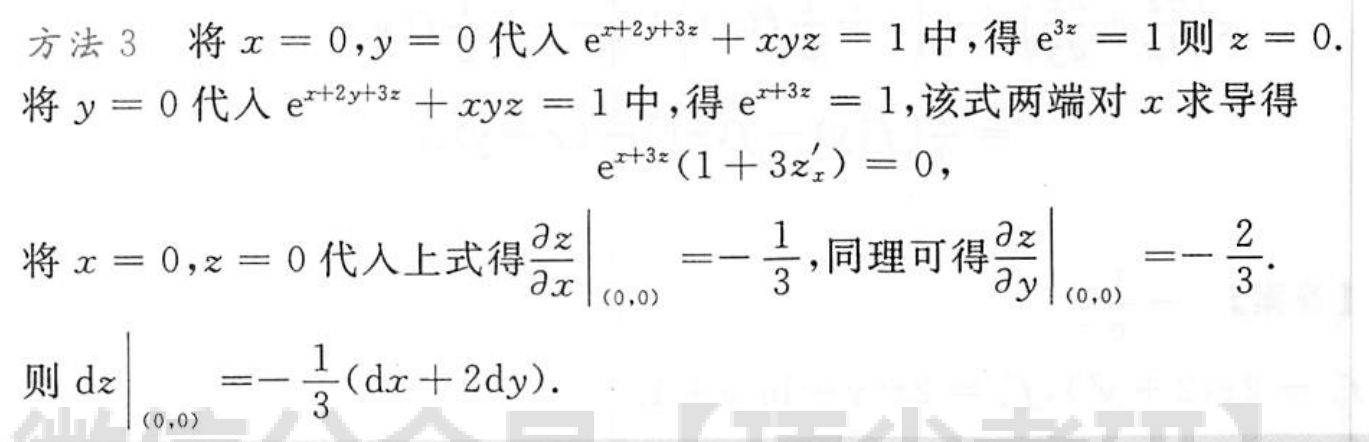多元函数求极值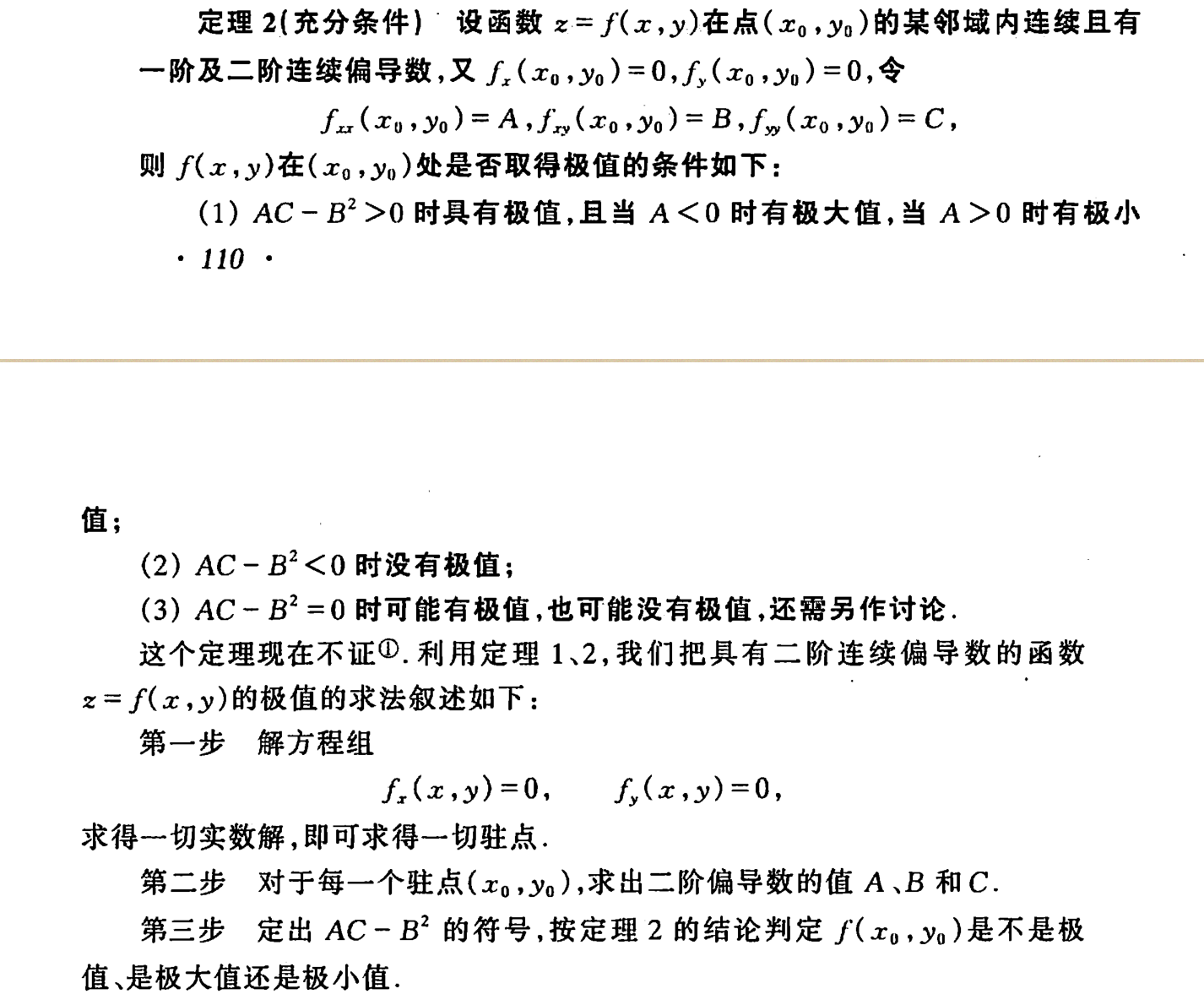交换积分次序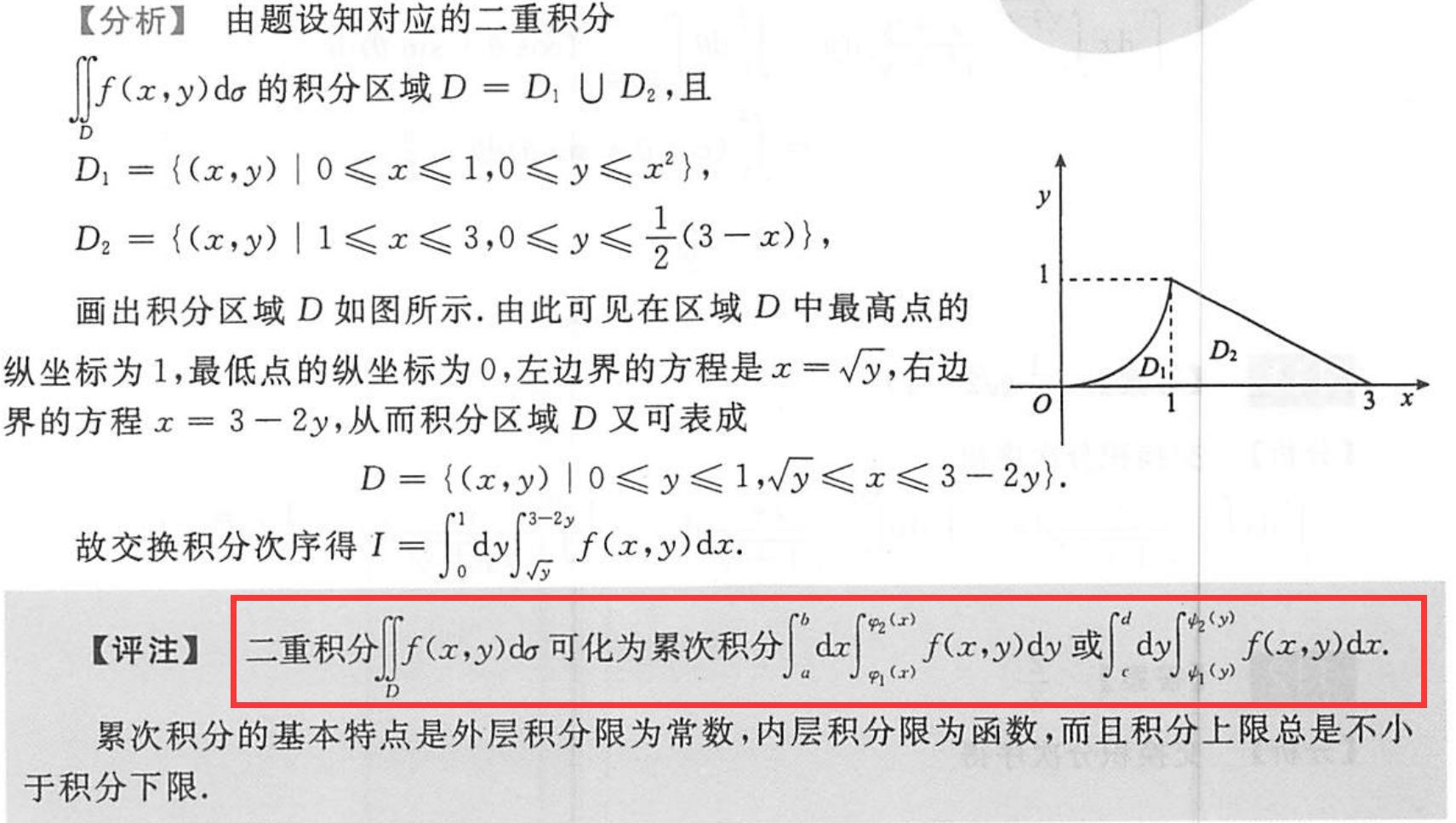展开全文线性代数
• 一、隐函数的定义： 一般的，如果在方程F（x,y）=0中，x取某区间内任一值时，相应地总有满足这方程唯一的y值存在，那么就说方程F（x,y）=0在该区间内确定了一个...二、隐函数如何求导： 即：（1）对式子中的x求导 (...
一、隐函数的定义： 一般的，如果在方程F（x,y）=0中，x取某区间内任一值时，相应地总有满足这方程唯一的y值存在，那么就说方程F（x,y）=0在该区间内确定了一个隐函数。
即：在方程F（x,y）=0中，能确定y是x的函数的就是隐函数（函数是指在某一变化过程中两个变量x、y，对于某一范围的x的每一个值，y都有确定的值和它对应，y就是x的函数）
二、隐函数如何求导：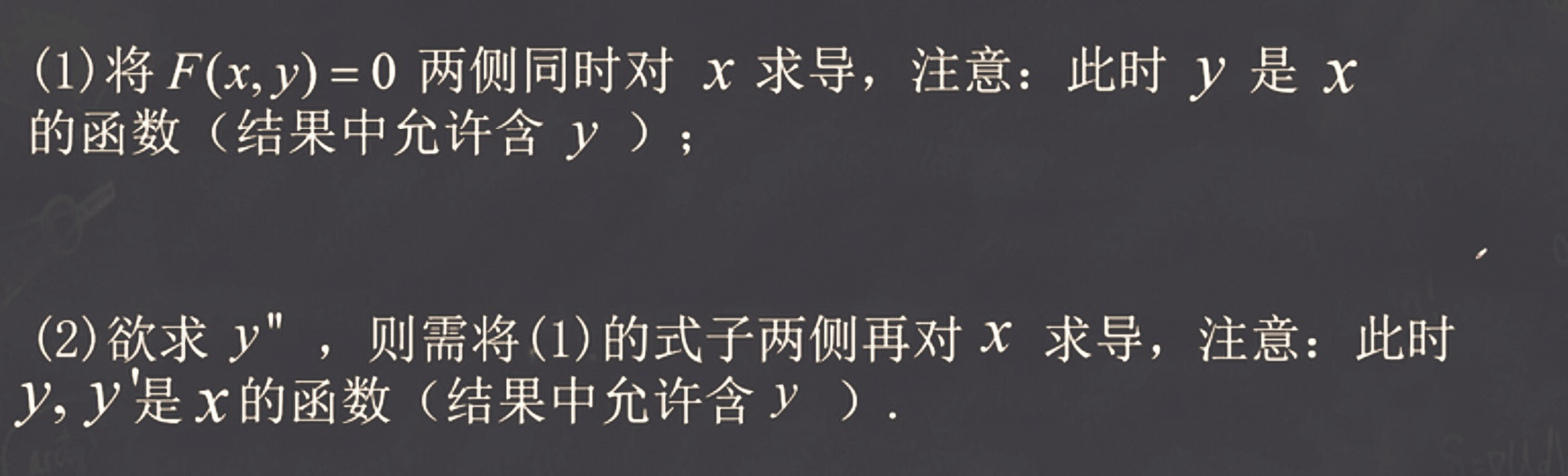即：（1）对式子中的x求导 (2)将式子中的y看做一个关于x的式子，所以要对x这个式子求导，即对y这一整体求导，写作dy/dx 或y’.
-----------------------------------------------------------------习题------ 1、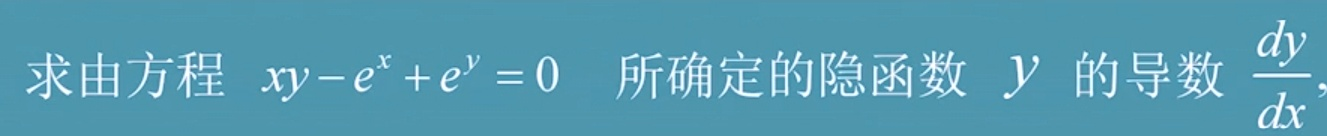两边对x求导：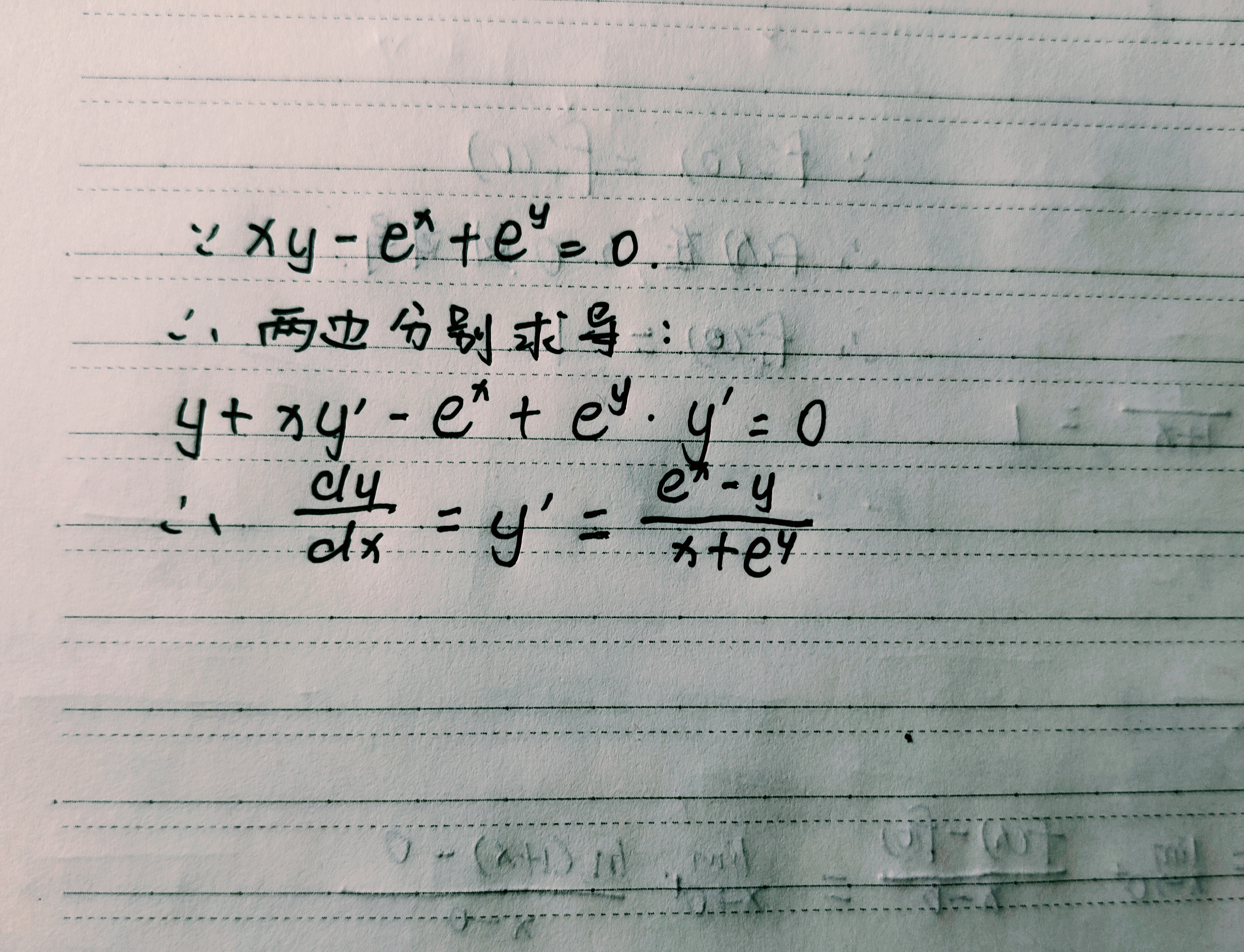最后由于求的是y’,要通过整理式子得出。
2、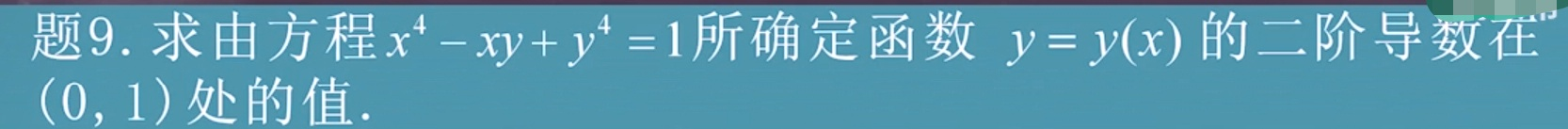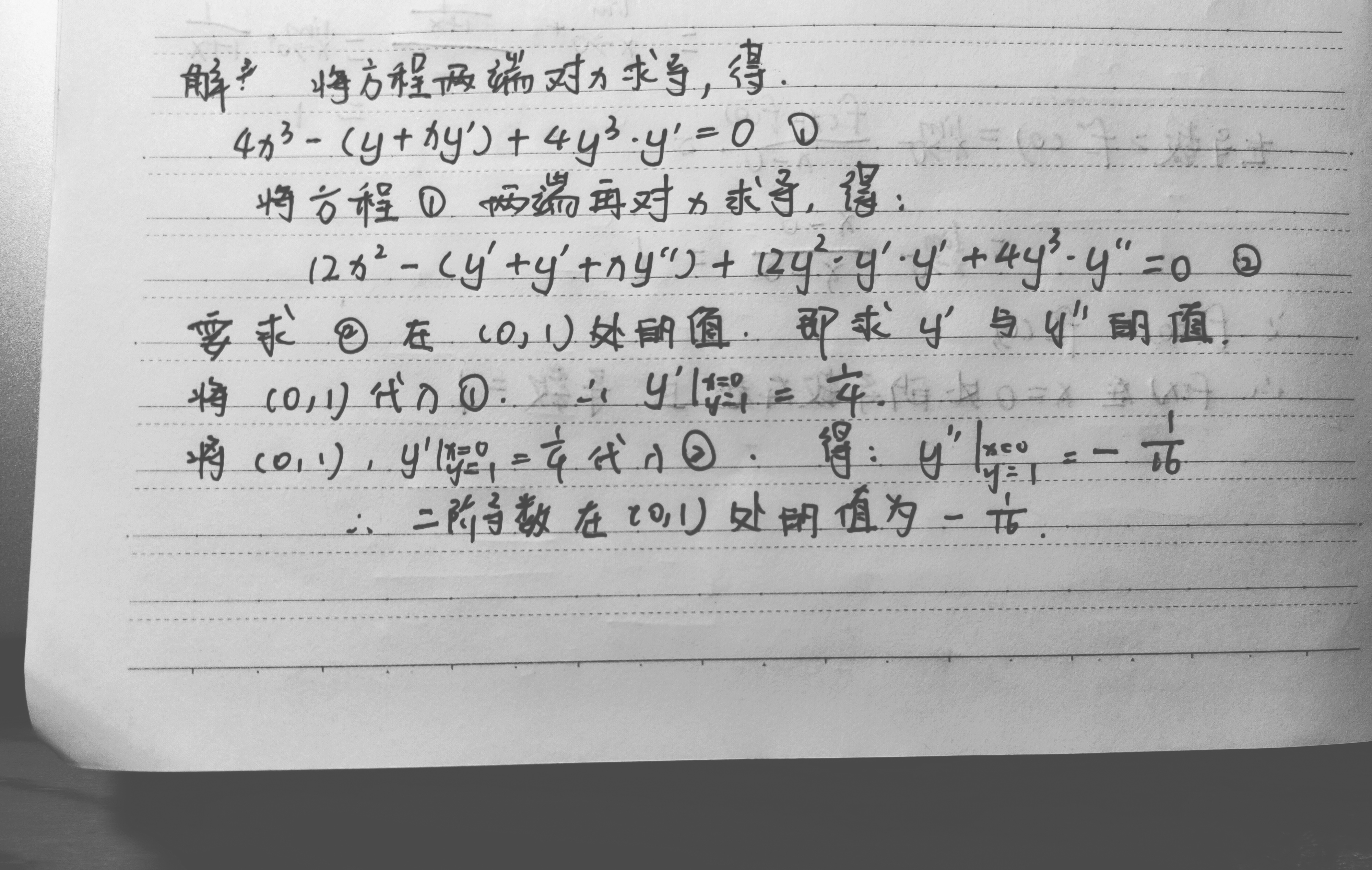展开全文• §8.5 隐函数求导公式 一、二元方程所确定的隐函数的情形 由二元方程可确定一个一元的隐函数,将之代入原方程,得到一个恒等式 对恒等式两边关于变量求导,左边是多元复合函数,它对变量的导数为 右边的导数...
• 隐函数求导——对等式方程两边同时求导具有怎样的实际意义呢？
• 过椭圆x2a2+y2b2=1上一点的切线方程为x0xa2+y0yb2=1y′=−b2xa2y,则斜率k=−b2x0a2y0切线方程y−y0=−b2x0a2y0（x−x0）带入x02a2+y02b2=1，则x0xa2+y0yb2=1 过椭圆\frac{x^2}{a^2}+\frac{y^2}{b^2}=1上一点的切线...
• 1.由一个方程式确定的隐函数（一元函数）求导法 设F(x,y)有连续一阶偏导数，且Fy'!=0，且由方程确定的函数y=y(x)可导，则 2.由一个方程式确定的隐函数（二元函数）求导法 设F(x,y,z)有连续一阶偏导数，且Fz'!=0...
• ## 高数——隐函数与参数方程求导

千次阅读 多人点赞 2019-10-18 11:19:16
隐函数 如果方程f(x,y)=0能确定y是x的函数，那么称这种方式表示的函数是隐函数。而函数就是指：在某一变化过程中，两个变量x、y，对于某一范围内的x的每一个值，y都有确定的值和它对应，y就是x的函数。这种关系一般...
• 现有时间和路程的函数关系 S=S(t)，质点在时间点t的速度为v(t)=S’(t)=dS/dt,那么质点在时间点t的加速度呢？就是质点在时间点t的速度的变化率，a(t)=v’(t)=d(dS/dt)/dt 注解 以上就是高阶导数的引入内容，二阶...
• 隐函数求导结果是这样的？但是为啥呢？ 开始探究 1. 相关变化率 考虑一个梯子长5米 问如果梯子以1m/s下滑，问你梯子底端的移动速度多少？ 我们定义y(t)和x(t)的函数，表示这两段随时间的变化。说实话我...
• 在说到隐函数(Implicit function)之前，先回想一下显函数(Explicit function).0.显函数(Explicit function)解析式中明显地用一个变量的代数式表示另一个变量时，称为显函数。即总能写成y=f(x)y=f(x)的形式。 1....
• 3.隐函数求导法 一：复合函数求导数与全微分 例题1：具体点先代后求、导数的定义 例题2：全微分 例题3：变上限 例题4:幂函数形式（多元复合） 例题5 例题6 例题7 例题8：含义抽象函数的复合函数求二阶偏导 ...
• ## 隐函数的求导

千次阅读 2019-07-05 15:02:53
今天上物理课时偶然想到了隐函数求导的严谨证明，那么就记一下吧。 首先，如果我们要对一个隐函数求导，我们首先需要证明这个隐函数连续可微 以及，由于我太菜了，所以本文的隐函数只对于二维的隐函数进行了讨论 ...
• 高等数学电子同济六隐函数求导公式PPT课件.pptx
• 隐函数求导3.对数求导4.参数函数求导 1. 利用莱布尼茨定理求高阶导 只看两点： 1、常用导数的高阶公式 2、例题 例题： 2.隐函数求导 这种方程里面y是x的函数，但是不显性。 例题1 设 y=y(x),y2−2xy+9=0y = y(x), ...
• 什么是隐函数？ 如果方程F(x,y)=0能确定y是x的函数，那么称这种方式表示的函数是隐函数。 本质上F(x,y)=0函数y=f(x)是一样的，但是在数学理论中，总有一些函数，人们已经证明它们的函数关系，但是还是无法表示成显...
• 本文介绍了利用隐函数、对数、以及参数方程求导的概念和方法。人工智能 数学 参数方程
• 本章主要讲解隐函数求导和相关变化率，大家理解什么叫隐函数及其如何求导 隐函数求导 相关变化率 隐函数求导 首先我们来理清什么叫隐函数，讲解隐函数之前我们来讲显函数，因为隐函数是相对显函数而言的 ...
•数学
• 数学 - 基本初等函数导数公式及求导法则 三角函数相关运算 ...隐函数求导 “如果方程F(x,y)=0能确定y是x的函数，那么称这种方式表示的函数是隐函数。一般情况下无法写成y=f(x)这种格式，任何的显函数...
• 求导公式 导数运输法则 复合函数求导法则 幂指函数求导 取对数后按照符合函数求导法则数学
• 1、一元函数与多元函数复合的情形 若函数u=ϕ(t)、v=ψ(t)u=ϕ(t)、v=ψ(t)u = \phi(t)、v = \psi(t)都在点ttt可导，函数z=f(u,v)z=f(u,v)z=f(u,v)在对应点(u,v)(u,v)(u,v)具有连续偏导数，那么复合函数z=f[ϕ(t),...高等数学
• §8.4 多元函数求导法则 【定理】若函数及都在点可导; 函数在对应点具有连续偏导数, 则复合函数在点可导,且其导数为  (1) 证明:设获得增量,这时的对应增量为,函数的对应增量为。 据假定,函数在点具有连续偏导数,...高等数学 复合函数
• 隐函数求导3.对数求导4.参数函数求导5.用导数求切线、法线6.函数的微分1. 利用莱布尼茨定理求高阶导只看两点： 1、常用导数的高阶公式2、例题例题2.隐函数求导这种方程里面y是x的函数，但是不显性。例题1 设 求 解：...
• 转载于:https://www.cnblogs.com/tszr/p/11168079.html
• 微积分的核心是极限(Limit)，求导(Derivative)是微积分的重要内容，本质...所以本文想系统的梳理一下求导法则及常见函数求导公式，争取利用最少的知识把下面公式都推导出来。图：常见函数求导公式一、导数的定义AB......